## Sunday, September 30, 2012

### Honnold-Mudd Library and The Odds of Winning the World Series

Greetings everyone!

Honnold Mudd Library

Yesterday I visited the Honnold Mudd Library, which is part of the libraries of the Claremont Colleges. The library has open for most of the day and has room for the public. Amazingly, this is the first collegiate library that I have ever been to where the mathematics section is located on the first floor. The bookshelves for this section are on rollers - which allowed one aisle to be open at a time.

I spent the better part of four hours there, reading about game theory, probability, and the Zeta function.

Here is the link to the Claremont College Libraries' web page.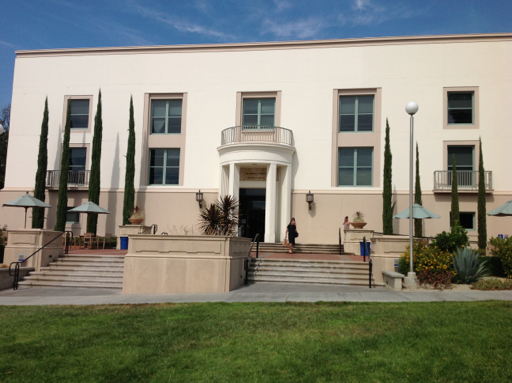The Odds of Winning the World Series

Every October in the United States (and Canada should the Toronto Blue Jays be one of the contenders), Major League Baseball (a.k.a. MLB) has an annual tournament of 10 teams to determine the World Series winner. The 10 teams consists of six division winners and four "wild-card" teams. MLB started the current format this year.

Out of the 10 teams, 2 final teams, the National League Champion and American League Champion meet for the World Series. The series is a best of seven series, where the first team to win four games wins the Series and is the Championship team for that year.

We can calculate the odds of a team winning that series by using the Binomial distribution, with the following formula:

nCr × p^r × (1-p)^(n-r)

Where:
n = number of trials
r = number of successes
p = probability of success of one trail
1 - p = q = probability of failure of one trail. Note that p + q = 1.
nCr = combination of n objects taken r objects at a time = n! ÷ ((n - r)! r!)

Even Odds

Let's say two teams are evenly matched. That is, p = 0.50, and q = 1 - p = 0.50. What will be the odds whether one team wins the World Series in four games? Five games? Six games? Seven games?

Win the Series in Four Games

If a team wins a game in four games, it is said that the victorious team swept the losing team. To achieve the probability of a sweep, find the combination of winning all four games and multiply by the odds. That is:

4C4 × 0.50^4 × 0.50^0 = 1 × 0.50^4 = 0.0625

Winning the Series in Five Games

In order to win the series in five games, you must win three games and lose one in the first four games. Obviously, you have to win the fifth game. In calculating probability for this situation, it really does not matter which order you win the first three in four, but that it happens. The "lack of order" requirement allows us to use combinations.

Multiply the result of winning 3 out of 4 games by the odds of winning the fifth game to get the total probability. The total probability of winning the World Series in five games is:

P(winning 3 games out of 4) × P(winning the fifth game)
(4C3 × 0.50^3 × 0.50^1) × (0.50)
= 0.25 × 0.50
= 0.125

Winning the Series in Six Games

In order to win the series in six games, you must win three games and lose two in the first five games. Using a similar approach to the 5 game situation, the total probability of winning the World Series in six games is:

P(winning 3 games out of 5) × P(winning the sixth game)
(5C3 × 0.50^3 × 0.50^2) × 0.50
= 0.3125 × 0.50
= 0.15625

Winning the Series in Seven Games

Similarly, in order to win the series in seven games, you and your opponent win three games apiece in the first six games. Using a similar approach to the 6. game situation, the total probability of winning the World Series in seven games is:

P(winning 3 games out of 6) × P(winning the seventh game)
(6C3 × 0.50^3 × 0.50^3) × 0.50
= 0.3125 × 0.50
= 0.15625

Not surprisingly, the odds of your team winning the World Series in an evenly matched series is 50%. (0.0625 + 0.125 + 0.15625 + 0.15625 = 0.50.)

Underdogs and Favorites

What if the teams are not evenly matched? Instead, we have an underdog, whose odds of winning each game is less than 50%; and a favorite, whose odds of winning each game is greater than 50%. We can use the analysis presented above to calculate the odds of winning the World Series.

For example, say the National League Champion San Francisco Giants, the underdog, plays against the American League Champion New York Yankees. Expert odds makers estimate that the Yankees win about 55% of their games against the Giants. What are the odds of each team winning the World Series?

From the Giants' Point of View, the odds of winning a game is 45%, or p = 0.45. (q = 0.55) Remember each team can win the World Series in one of four ways: sweep (in four games), in 5 games, in 6 games, and in 7 games.

Sweep:
P(win 4 games of 4) = 4C4 × 0.45^4 × 0.55^0 ≈ 0.0410063

Win in 5 Games:
P(win 3 games of 4) × P(winning the 5th game)
= (4C3 × 0.45^3 × 0.55^1) × 0.45 ≈ 0.0902138

Win in 6 Games:
P(win 3 of 5 games) × P(winning the 6th game)
= (5C3 × 0.45^3 × 0.55^2) × 0.45 ≈ 0.1240439

Win in 7 Games:
P(win 3 of 6 games) × P(winning the 7th game)
= (6C3 × 0.45^3 × 0.55^3) × 0.45 ≈ 0.1364483

Total Chance of the Giants winning the World Series (with p = 0.45):
0.0410063 + 0.0902138 + 0.1240439 + 0.1364483 = 0.3917122 (approximately 39.17%)

Going to the Yankees, the favorites in this example. Now the probabilities are reversed, with p = 0.55 (q = 0.45).

Sweep:
P(win 4 games of 4) = 4C4 × 0.55^4 × 0.45^0 ≈ 0.0915063

Win in 5 Games:
P(win 3 games of 4) × P(winning the 5th game)
= (4C3 × 0.55^3 × 0.45^1) × 0.55 ≈ 0.1647113

Win in 6 Games:
P(win 3 of 5 games) × P(winning the 6th game)
= (5C3 × 0.55^3 × 0.45^2) × 0.55 ≈ 0.1853002

Win in 7 Games:
P(win 3 of 6 games) × P(winning the 7th game)
= (6C3 × 0.55^3 × 0.45^3) × 0.55 ≈ 0.1667701

Total Chance of the Yankees winning the World Series, with p = 0.55,
0.0915063 + 0.1647113 + 0.1853002 + 0.1667701 = 0.6082878 (approximately 60.83%)

Note: 0.3917122 + 0.6082878 = 1. As a check, see if the total probabilities add to 1. Being a favorite can significantly increase your chance of winning the World Series!

A RPN Program (HP 15C)

Here is a RPN (Reverse Polish Notation) keystroke program to calculate

nCr × p^r × (1-p)^(n-r)

I used a HP 15C. On the 15C (and 32S, 35S), recall arithmetic can be used to save space. Adjust accordingly.

(Key: Key Code)
LBL A: 42 21 11
RCL 3: 45 3
RCL 2: 45 2
y^x: 14
1: 1
RCL- 3: 45 30 3
RCL 1: 45 1
RCL- 2: 45 30 2
y^x: 14
×: 20
RCL 1: 45 1
RCL 2: 45 2
Cy,x: 43 40
×: 20
RTN: 43 32
(15 steps)

Input:
Preload the following memory registers: R1 = n, R2 = r, and R3 = p

Output:
Probability on the x stack level (display), with the previous contents of the x stack level on the y, z, and t stack levels.

Source of the formula: Packel, Edward. "The Mathematics of Games and Gambling". MAA 1981. New York

Before I go for today, I leave you with several fun facts:

Let z be any complex number, where z = a + bi, with a the real component and b the imaginary part

The Riemann Zeta Function has no zeros (roots) when a is greater than 1.

The Gamma Function has no zeros when a is greater than 0.

Source: Patterson, S.J. "An Introduction to the Theory of the Riemann Zeta Function" Cambridge University Press 1988.

This blog is property of Edward Shore, 2012.

## Monday, September 24, 2012

### iOS App Review: PowerOne FE - 9/24/2012

As we move closer to using our tablets, smartphones, and other "one-stop" machines for literally everything, I want to start reviewing calculator apps on occasion. Today, it is the PowerOne App. All the screen shots are from the iPad app.

App: PowerOne
Software created by Infinity Softworks
Type of Calculator: Finance, Scientific, Construction, among possibly others
Are there Lite editions? Yes
Price of the Full Edition: \$4.99 (Update 9/15/2012)
Available on: iPhone/iPod Touch, iPad (I do not know if an Android version is available)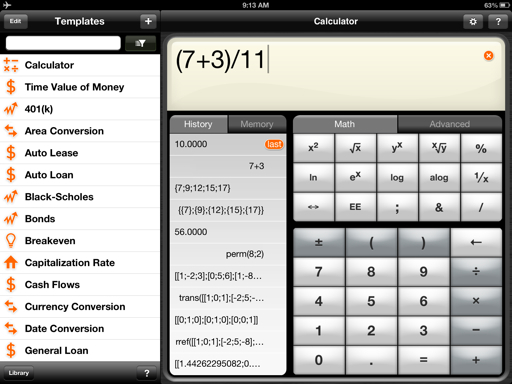Features:

* Full force scientific calculator with calculus, matrices, vectors, complex numbers, and basic logic computations
* Templates for every kind of application. They are easily accessed in with the Library app.
* You have the ability to create your own templates and share them via email. You can also share results from templates.
* The calculator works in either Algebraic or RPN mode.

Pros:

* Templates are available for most applications. You can share results and templates through email.
* You can mark templates as favorites and organize them.
* Often, the templates will self-calculate results as you enter data.
* The version you purchase will pre-load templates from appropriate subject. However, with any version, you can access all templates. I am running the Finance version.
* The factorial function (fact) works for all real arguments.
* Complex functionality extends to the trigonometric functions and exponential functions.

Four examples of templates are shown below: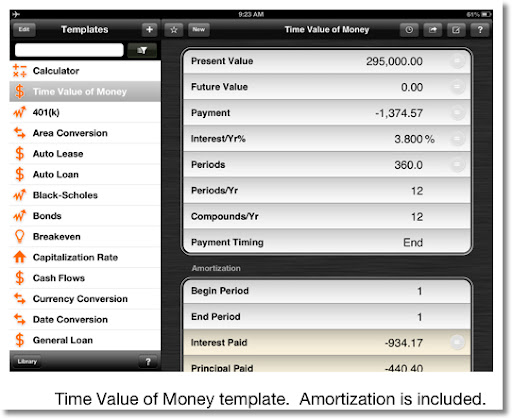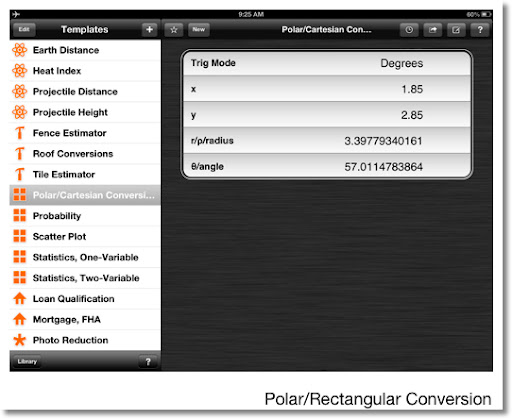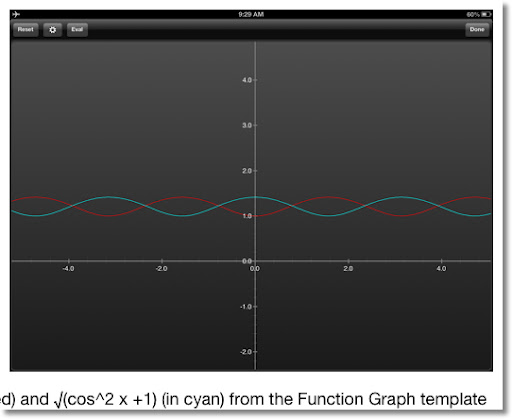Cons:

* The calculator is very cumbersome to use if you want to use advanced functions. First, you have to select different keyboards (trigonometry, number, etc) to get access to different commands. The Advance tab gets you access to these keyboards.
* The Help button gives only basics, and but syntax for each function. If you want syntax, you have to call up the Advanced Tab, pressing more, and scrolling down. Don't forget if you want to access the different keyboards, you have to scroll back up.
* The language is cumbersome. I have not tried to program a template in this version in the app, but the learning curve seems to be steep.
* If you want statistical functions, use the templates rather than the calculator, because the functions are confusing to get to run properly.
* Regarding the Function Graph template, you will need to type the entire function yourself. No access to the function calls. Worse, the keyboard does not stay on the alphabetic keyboard, and inconsistently switches back to the numeric keyboard. Almost impossible to type functions. Note: This complaint is limited to the iPad. Thankfully the iPhone/iPod Touch version does not have this problem.
* When entering arguments in templates, the numeric keypad shows up with no functionality. The arithmetic functions show up by the press of the Math key, but it blocks your number pad! Also, no way to enter π other than typing the value out: 3.14159265359... (See below)

Overall:

* If you are looking for solvers to common problems, this app is great for it. Many templates are available.
* For this version (I can't find the version number at this time), I would not recommend this app for regular calculator use, at least the iPad version. The iPhone version may be more useable as far as the calculator is concerned.

Syntax of some functions and numbers are presented here. Spaces between arguments are added for readability only; not required.

Any principal root: root(y; x) returns y^(1/x)

Complex Numbers - rectangular: (real ; imag)

Complex Numbers - polar: (radius ;@ angle). The Help screen got the syntax wrong, you need both the color and at sign.

Base Designations: _d, _b, _o, _h (but Hexadecimal numbers must start with a number) are entered by pressing the dec, bin, oct, and hex keys on the Programmer keyboard, respectively.

Matrices: [ [a_11 ; a_12 ; a_13 ; ... ] ; [a_21 ; ...] ... ]. Colons separate row entries as well as columns.

Numeric Derivative: nDeriv("f(x)" ; "x" ;point). The function and its variable are enclosed in quotes. The quotes are included on the Calculus keypad.

Numeric Integral: fnInt("f(x)" ; "x" ; lower limit ; upper limit). The function and variable are enclosed in quotes.

In the Statistics keyboard, the nCr and nPr keys calls up the comb and perm functions, respectively. Both take two arguments, n and r.

As always, comments and questions are welcome.

Until next time,
Eddie

This blog is property of Edward Shore. © 2012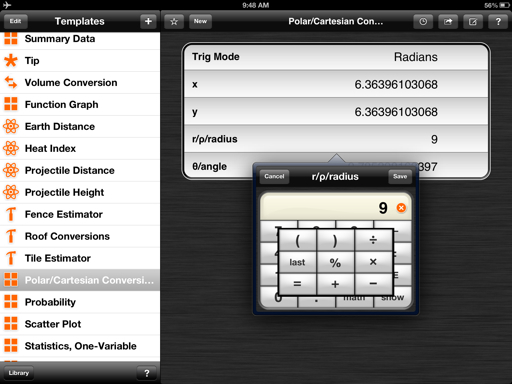### HHC 2012 Day 2 Summary - 9/23/2012

Here is what went on during Day 2 of the HHC Conference:

Wlodek Mier-Jedzejowicz - "Is It a Really Pathetic Name." (My apologizes in advance if I misspell the names)
* RPN (Reverse Polish Notation) came to be by Polish mathematician and logician Jan Łukasiewicz. Łukasiewicz was also a philosopher, teacher, and worked with multi-value logic.
* Mier-Jedzejowicz's family has connections with Łukasiewicz: he was a professor to several of his relatives.
* At the 10th Anniversary of the HPCC (based in Great Britian), Dr. William Wickes wrote an article in "RCL 20: People, Dreams, & HP Calculators" about the naming of RPN. Dr. Wickes was not too happy with the name (see the title for the hint).
* Although several alternative names were given, including "natural operating system". Mier-Jedzejowicz defended the RPN name.

An example of notations: Say we want to calculate 7 + 11.
Infix or algebraic notation (TI, Casio, and most calculators): 7 + 11
Polish notation: + 7 11
Reverse Polish Notation (HP): 7 11 +

Namir Shammas - "Variable Sampling Integration"
* The program has first written on the HP 41.
* The main difference is that the change in x (often labeled "h" or "Δx") is not constant. Where the intervals are placed depends on the slope of f(x). More intervals are placed when f(x) is changing quickly.
* Smaller areas are calculated using the area under a quadratic or cubic curve and then aggregated.
* In general, variable sample integration is more accurate than Simpson's rule when using cubic polynomials to calculate areas of sub-intervals. However, Simpson's rule is better when f(x) contains trigonometric functions.

Rick Furr - "The Making of the HPV2 Poster"
* Furr is an avid collector of calculators: over 1,000 of them. The collection includes Curta mechanical calculators.
* The HP calculator poster is an update to 2005 poster. I purchased one and will be posting a picture of it.
* Furr produced several posters: one covering a Cuerta machine and its hundreds of parts, the history of telephones, and the history of money, specifically greenbacks.
* The process of the new poster in a nutshell:
1. Take a picture of each calculator. Furr commented that inspection lights work best. Also take the picture away from the calculator and use the camera's zoom features.
2. Furr used the desktop publishing software Serif PagePlus x5 to plan the poster. This preferable to Photoshop since images can be scaled while the resolution is preserved.
3. Find data for each calculator and peripheral. This proved to be a challenge.
4. Use a printer that uses a stochastic printing process. The random grouping of the dots (cyan, magenta, yellow, black) allows for sharper printing.

As soon as I can will post a picture of the HP poster, probably when I get back home.

Rick Furr's website: http://www.vcalc.net/

Jakie Waldering - Raspberry Pi
* Waldering showed the Raspberry Pi, a small computer that runs on Linux. How small is it? It can fit in your hand!
* Some specifications:
The computer boots from a 1G SD Card
The computer has an Ethernet port and two USB ports
It has an HDMI connection, so the computer can run on high-tech televisions
Graphics: 48 GPU Cores - screen refreshes at 40 to 50 screens per second
Does not get not after use - which was discovered after running a network of six Raspberry Pis ran a local network. The students were playing Quake 3.
This is a low cost device. Some assembly required.

Final Thoughts
* I always have so such fun at the HHC conferences. If you get a chance, go. The HHC is an annual conference will be about 9/21/2013. Place to be determined.

Web Pages:
Gene Wright: http://www.rskey.org/gene/hpgene/
HPcalc.org, run by Eric Rechlin: http://www.hpcalc.org/. Rechlin also makes the keyboard stickers for the WP 34S.

I leave you with some pictures from the conference.

Eddie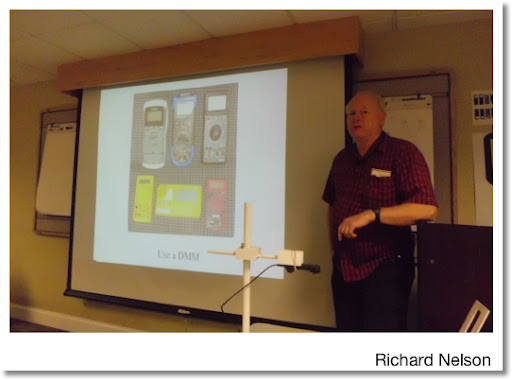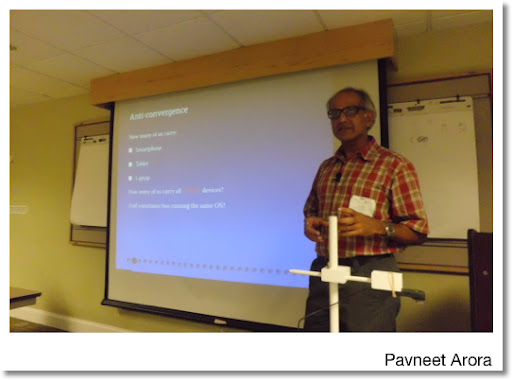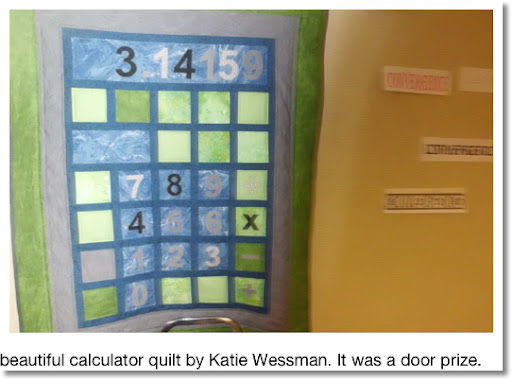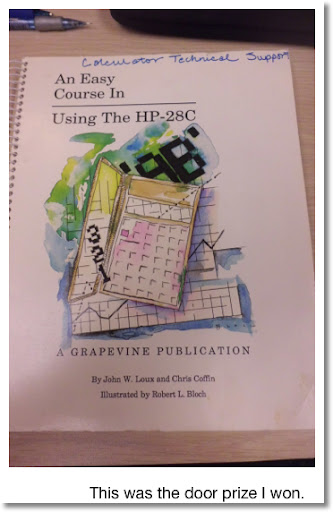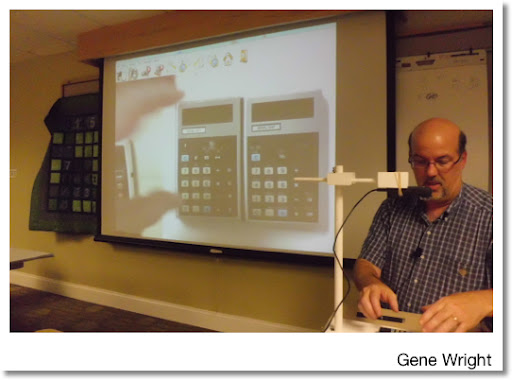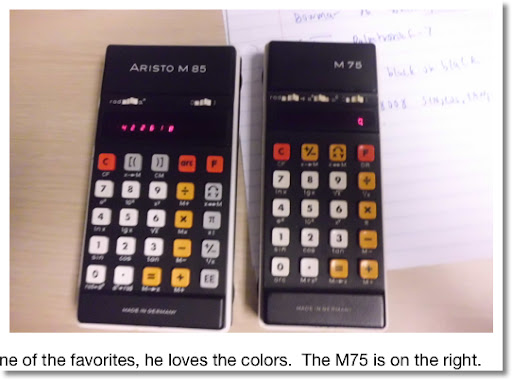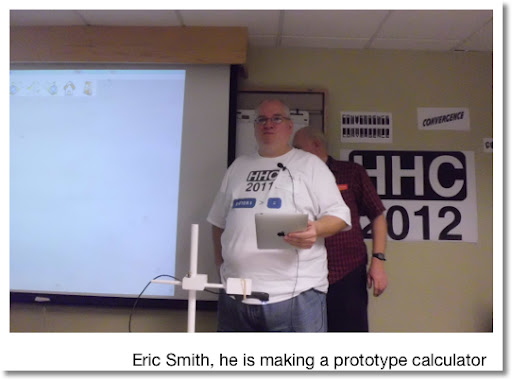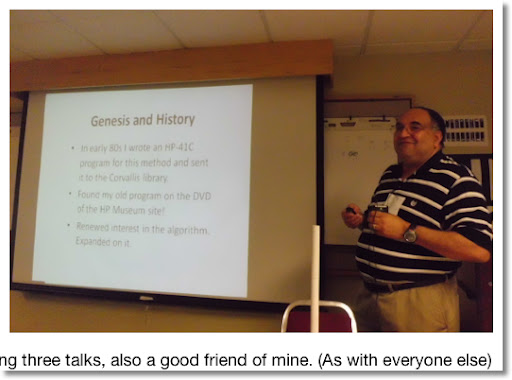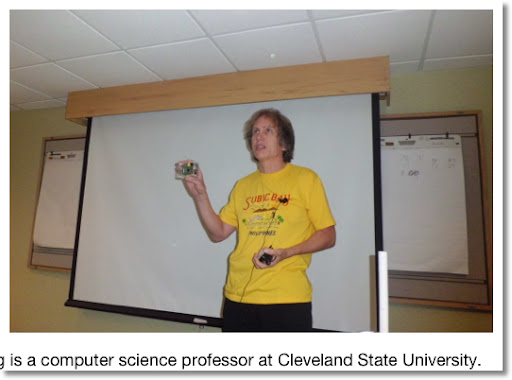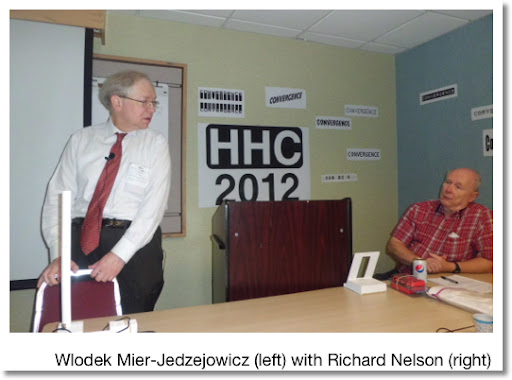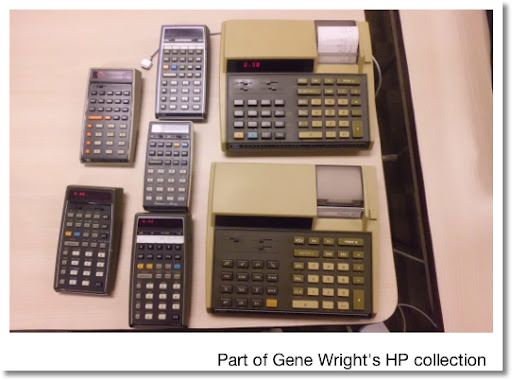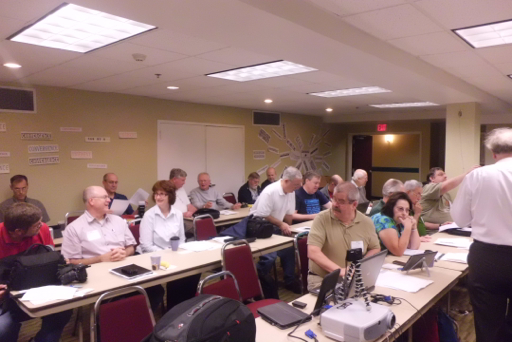## Sunday, September 23, 2012

### HHC 2012 Day 1 Summary - 9/22/2012

Greetings from Nashville!

I am at the HHC 12 conference. It is an annual conference for Hewlett Packard Calculator enthusiasts, held every September. This has been my fourth conference I attended. Everyone that I meet at the conference is super. I love going to these conferences. Here is a summary of went on during the 13-plus hour day, which honestly, went by amazingly fast.

Before the conference started, I was privileged to purchase a HP 32s II calculator from Bob Patton. A picture of it is shown below. The HP 32s II is a highly sought RPN programmable calculator that was in production during most of the 1990s.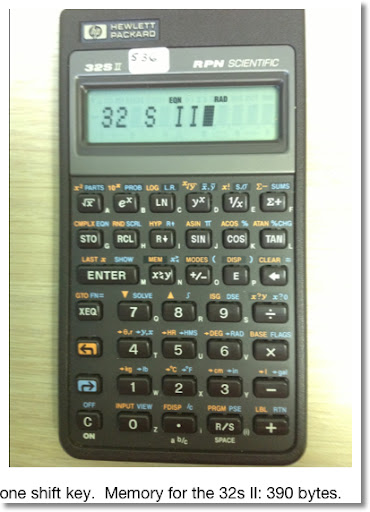Today's conference started at the bright and early time at 8:15 AM. Here is a short summary:

"WP-34S and the HP Calculator Way" - Jake Schwartz:
* In the 1970s, Hewlett Packard (from here on in will be referred to as HP), was wowing calculator owners by constantly exceeding expectations. This due to the fact that handheld electronic calculators were relatively new.
* Around the time that the HP 49g+ was introduced in 2003, HP and calculator users crossed a point. Now, we users are suggesting more features to HP than HP has produced. This resulted in some users making home made calculators by repurposing them. For example, the HP 20B and 30b are repurposed to become a scientific programming calculator, the WP 34S, despite working with limitations of memory, manual keyboard labeling, and limited screen.
* Today, there is less documentation. Also calculators have been aimed towards certain niche markets, primarily education.

"Tablets vs. HP Calculators" - Gene Wright
* Of a poll of 41 attendees: 18 have tablets. 8 of the tablet have Apple tablets, including me. In fact, I write my blog with my iPad, using the Blogger+ app. 24 of 41 attendees use smart phones.
* We are going towards a digital world. Textbooks are now being converts to PDF files for use on tablets. The university I work for, Cal Poly Pomona, has just participated in an e-book pilot program.
* The majority of young students now have access to tablets and smartphones. A concern among teachers is the issue of cheating with these devices. That has not stopped some schools from standardizing tablet use, such as the iPad.
* There is a consensus that the use of stand alone technology, such as cameras, physical calculators, and GPS devices, is shrinking in favor of do-everything tablets.
* Jakie Woldering, a computer science professor at Cleveland State University, commented that we bring skills that will help us transition from one technology to the other.

"Calculator Root Finding: Scan Range Method" - Namir Shammas
* This program finds root, extrema (function minimums and maximums), and inflection points of f(x), using a Laguerre method.
* The user gives a domain to find points of interest of f(x) using a step size and other criteria.
* More information can be found here. Check under the HHC 2012 section.

"Measuring HP Calculator Current" - Richard Nelson
* Richard Nelson demonstrates how to find current and voltage of calculators in four states: off, during boot up, on stand by, and running a calculation. This allows us a way to determine if the keyboard and microprocessor are working.

"Calculating Before Calculators" - Palmer Hanson
* Palmer Hanson brought machines pre-dating handheld calculators. Among them were abacuses, slide rules of every kind, a circular percentage wheel, and a three-arm protractor used for navigation.

"The HP-41 is Alive and Well" - Warren Farrow
* Warren Farrow recounted his story about how he purchased the HP-41. He runs a website, www.hp41.org, a rich resource for the HP 41 calculator.

"Where Can You Find the History of Calculator Applications?" - Felix Gross
* Felix Gross shows that we have so much information regarding calculators including books, hardware manuals, journals, and application journals. However, the last category lacks an organized system of archive. The applications range from finance, cattle herd composition, optimizing factors for heat transfer, map projection, and education.
* The peak for calculator documentation occurred around 1977-1984.
* Today's resources include NASA.gov and eric.ed.gov.
* Currently, he is looking for articles concerning finance, engineering, and documentation in foreign languages.

"Re-tooling the Calculator Tool" - Pavneet Arora
* Pavneet Arora makes a case that we are not merging towards one do-everything tablet. On the filed, there is still a need for specialized and durable tools.
* Tablets are not the best for rugged conditions. A problem also is present on relying on only one tool, with the risk of failure. Multiple tools that can handle a similar function still serves a purpose. In my opinion, it is good to have a backup device.
* Arora favors the use of watches and belts that can be used on the arm. In addition, he states that a smaller Kindle Touch, with its e-ink screen, can serve as a multi-use rugged device due to its operating software. E-ink is preferred over back-lit devices.
* He stresses that tool design must be appropriate for working conditions.

Katie Wessman quilted a lovely calculator quilt that will be given as a door prize.

Gene Wright brought and show some of his non-HP (and non-TI) collection, which was really neat. Among the collection is Royal 99T Scientific Calculator (1976), Royal 94F Business Calculator (1976), Unitrex SC30, Unitrex 90SC RPN Calculator (had a 12 digit display which is unheard of in the 1970s), Canon Palmtronic F-7, and several Commodore models including the N-60 (navigation based), S-61 (geared towards statistics), and M-55, which he let me borrow for the night. The weirdest one was the Sanyo CZ-8008, which had only five scientific functions: sine, cosine, tangent, π, and square root. Yep, no inverse trig functions. Gene, who had the Commodore calculators, acquired most of the others calculators from Scott Reynolds.

"Converting HP-41C Programs to PDF" - Jakie Woldering
* Jakie Woldering described he was able to convert HP 41C programs from the calculator and classic journals to a modern format (PDF). This was done by using wands to read magnetic storage cards, a lot of typing, and conversion software.

"Even Larger Scaled Reptiles of the Nordic Countries" - Eric Smith
* Eric Smith updated progress on a calculator he and his group is making. The most significant update is a bigger capacity RAM chip which allows a more complex operating system.

"Curve Fitting with Least Squares Relative Error" - Namir Shammas
* Namir discusses a curve fitting algorithm that minimizes relative error instead of absolute error.
* A result: when the range of data is small, the least-squares method with relative error is more accurate than using absolute error.

* Three dimensional vectors arithmetic, dot product, cross product
* Logarithmic Gamma Function (note the factorial function still only works for integers)
* Integration by discrete points
* Linear Regression
* Complex Number Arithmetic
* 2 × 2 matrices including arithmetic, inverses, and determinants
* Error function
* Bessel Function (a very rare preprogrammed function!)
* Poisson Distribution for distinct points
* Quadratic Formula including complex roots
* Gaussian Distribution (Normal Distribution) - lower tail area

The battery is rechargeable, and lasted up to three hours on a single charge. Amazingly, the manual can be found online.

Eddie

Day 2 to come!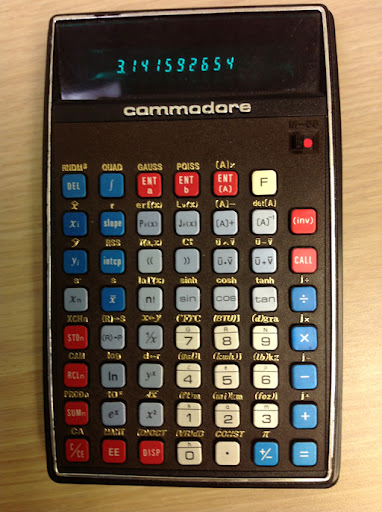## Tuesday, September 18, 2012

### Volume of a Parabolic Vase and Casio fx-78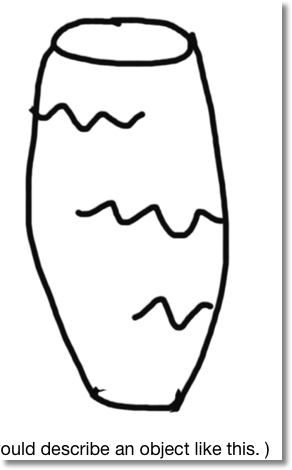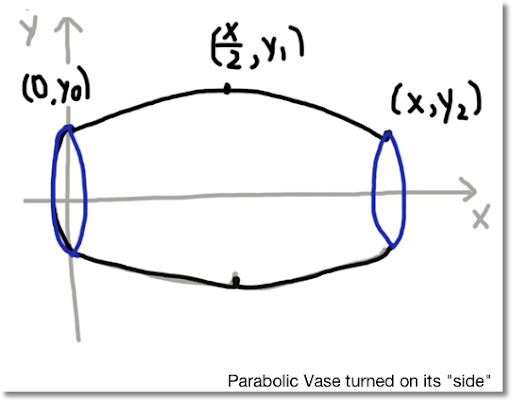Volume of a Parabolic Vase

Introduction

The following procedure is how to find the volume of a parabolic vase. This is a vase where the "sides" (for the lack of a better term) form a parabolic curve. Each side of a vase has a smooth bump, away from the center, with one peak. Imagine if that you can turn a vase on its side. The result is like the illustration above.

Note: The following procedure works with vases where the "sides" curved towards the center, rather than away.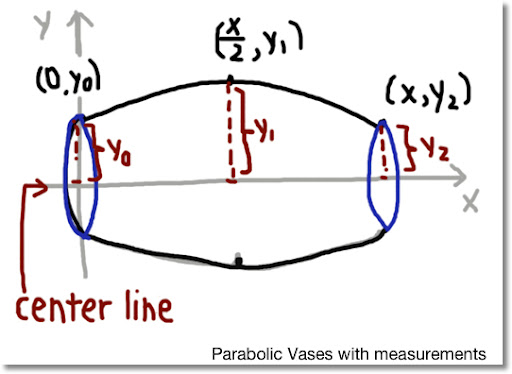Steps

Using calculus, we can approximate the the volume by taking the following steps:

1. Measure the length of the vase. Let x be this length.
2. Find the "center line" of the vase. From the center line, find the distance from the center line to the end of the case. Do this for both ends of the vase, and at half of the length. Get the best measurement you can. From one end to the other, these are lengths y_0, y_1, and y_2, respectively.
3. Use the data to form the parabolic curve. Find a, b, and c.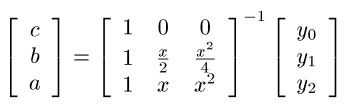4. With a, b, and c determined, plug in a, b, c, and x and calculate the following integral.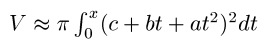Most graphing calculators can handle this task easily.

Note: If there is an official term for these type of vases, I would like to know. Many thanks in advance.

Example

Find the volume of a parabolic vase 4 inches long with the following: (see the illustration "Parabolic Vases with measurements").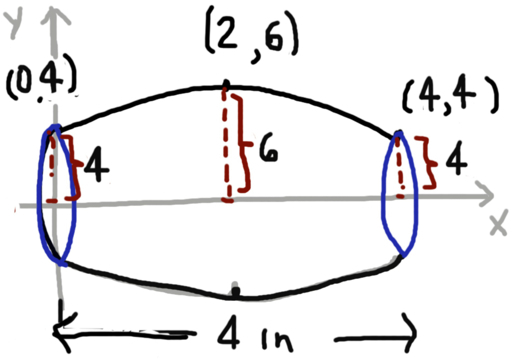So we have these points to form the parabolic equation with:
(0,4), (2,6), (4,4)

Then: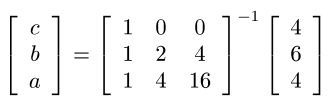c = 4
b = 2
a = -0.5

The integral is: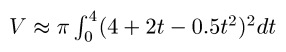Calculating the integral gives an approximate area of 134.041287 in^2.

Found at the Swap Meet: Casio fx-78

Several Sundays ago I purchased this calculator at the Azusa Swap Meet. This calculator, made in 1982, is not only basic, but quite small. It still works.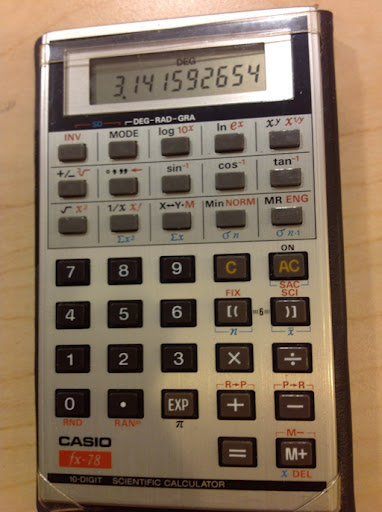Have a great day, Eddie

This blog is property of Edward Shore. © 2012

## Sunday, September 16, 2012

### Can't wait for Nashville!

I am going to a calculator conference in Nashville, TN next weekend. The conference is for Hewlett Packard calculator enthusiasts. Can't wait!

Eddie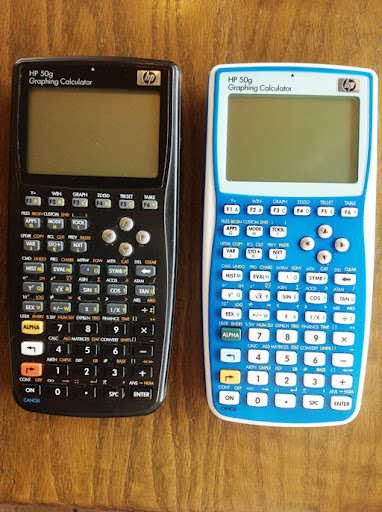## Thursday, September 13, 2012

### Cartesian Coordinates to Pixel (Screen) Coordinates

Converting Cartesian Coordinates to Screen Coordinates

When working with computer or calculator graphics, sometimes we have to work with screen coordinates. The screen coordinate system has the following:

1. The upper corner pixel (point) is (0,0).
2. The lower corner pixel is (A, B).
3. The x axis increases in the right direction.
4. The y axis increases in the down direction, the opposite direction of the Cartesian plane.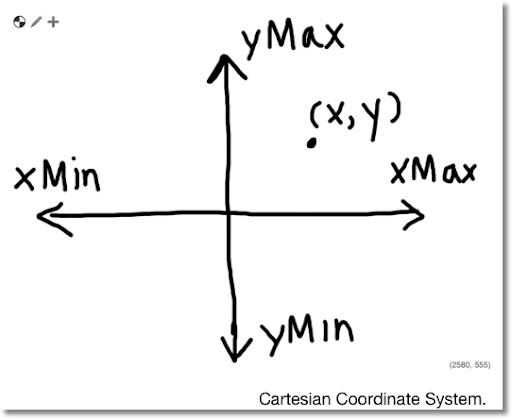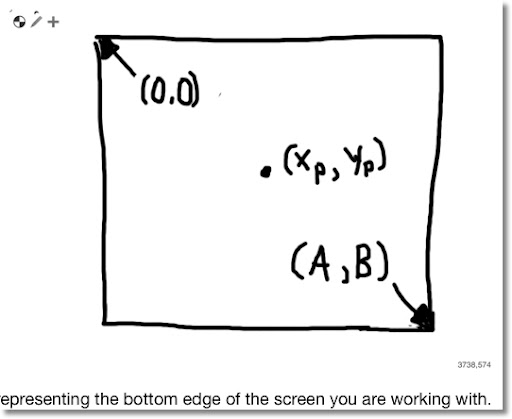In order to to convert a point (x, y) in the Cartesian coordinates to point (xp, yp) in Screen coordinates, first observe the following:

1. Picture the plane you are working with as a screen. If you are working with a calculator or computer, this is fairly easy.
2. Let Xmin be the least-valued x of the screen (left edge) and Xmax be the most-valued x (right edge). Similarly, let Ymin be the least-valued y of the screen (bottom) and Ymax be the most-valued y of the screen (top).

You can check with the window settings on a graphing calculator to verify Xmax, Xmin, Ymax, and Ymin.

For the Hewlett Packard 28 series, 48 series and 50g, the variables XRNG and YRNG list the screen's dimensions, {Xmin, Xmax} for XRNG and {Ymin, Ymax} for YRNG.

To transform Cartesian coordinates to screen coordinates, we can use transformation matrices for scaling and translation. The general formshow the transformation from coordinates (x, y) to (x', y') where:

s_x = scale of the new x axis. If s_x < 0, the x point is reflected with respective to the x axis.

s_y = scale of the new y axis. If s_y < 0, the y point is reflected with respected to the y axis. In going from Cartesian coordinates to screen coordinates, s_y < 0.

x_s = Is the translation (shift to the new center) in the x-direction. If x_s > 0, the new center is to the right of the original center. Similarly, if x_s < 0, the new center is to the left of the original center.

y_s = Is the translation (shift to the new center) in the y-direction. If y_s > 0, the new center is to the above the original center. Similarly, if y_s < 0, the new center is to the below the original center.

For the transformation from Cartesian coordinates to screen coordinates:

s_x = A / (Xmax - Xmin)

s_y = -B / (Ymax - Ymin)

Since the new center will be at (Xmin, Ymax):

x_s = -Xmin

y_s = -Ymax

Therefore by matrix multiplication, with x' = xp and y' = yp:

xp = (x - Xmin) * A / (Xmax - Xmin)

yp = (y - Ymax) * -B / (Ymax - Ymin)

On the HP 48 series and 50g, the translation from Cartesian to screen coordinates can be accomplished with the C→PX function.

Example:

Using the following window with settings Xmin = -5, Xmax = 5, Ymin = -4, and Ymax = 4, transform the Cartesian coordinate (1, 0) to screen coordinates. The screen has pixel size 130 × 79.

In this case, A = 130 and B = 79.

Then:

xp = 130 / (5 - (-5)) * (1 - (-5)) = 78
yp = -79 / (4 - (-4)) * (0 - 4) = 39.5

The screen coordinate of (1, 0) is {78, 39.5}.

HP 50g Programs

DPIX

This program figures the distance in pixels between two points stated in Cartesian coordinates.

Input:
2: coord-y
1: coord-x

Output:
1: Distance

Program:
<< C→PX SWAP C→PX - DUP * ΣLIST B→R √ R→B >>

Example:

Using the following window with settings Xmin = -5, Xmax = 5, Ymin = -4, and Ymax = 4, find the pixel distance between (0,1) and (-1,-2)? (HP 50g pixel dimension 130 × 79)

2: (0,1)
1: (-1,-2)
DPIX

Answer in Decimal mode: #32d. (Approxmiately 32)

ARCEZ

This draws the arc using Cartesian coordinates and the radius in Cartesian scale (the way one would think if drawing on a Cartesian plane). This program does all the necessary transformation for you. You can use it in a program (requiring a PICTURE command later in a program) or alone (press the left arrow button to show the graph screen).

Input:
4: Cartesian Coordinates (x,y)
3: Radius in Cartesian form (the way you think)
2: Beginning Angle
1: Ending Angle

<< → P R A B
<< P DUP R +
C→PX SWAP C→PX - DUP * ΣLIST B→R √ R→B
P C→PX SWAP A →NUM B →NUM ARC >> >>

Example:

Using the following window with settings Xmin = -5, Xmax = 5, Ymin = -4, and Ymax = 4, draw a circle with radius 1.5 and center (1,0). (HP 50g pixel dimension 130 × 79)

4: (1,0)
3: 1.5
2: 0
1: 2 * π
ARCEZ

Pressing the left arrow button will show the picture below (with no functions selected and additional drawing):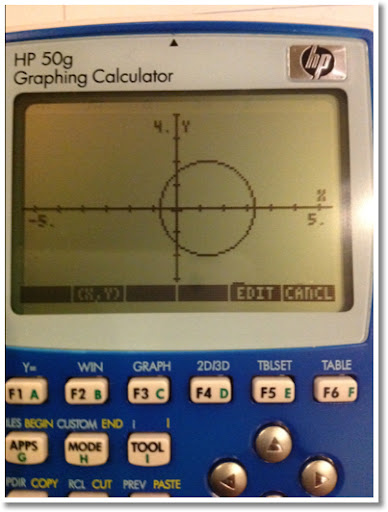This concludes today's blog entry. As always, thank you for all your comments and support. Have a great day!

This blog is property of Edward Shore. © 2012

## Tuesday, September 11, 2012

### September 11

Taking some time from my math blog to speak about today.

This is a day we in the United States will never forget. Blessings to the families who suffered the losses on that horrible day in 2001.

May this world find peace soon.

## Saturday, September 8, 2012

### Time Value of Money Tips and Tricks (Real Estate)

Tips and Tricks - Time Value of Money

Here is a way to solve the following financial problems with TVM (Time Value of Money) functions:

* Finding the balance of a loan
* Finding the total amount of interest paid
* Finding the APR
* Finding the maximum loan amount based on the 28/36 ratio

Conventions and Assumptions

Variables:
N = number of months
I/YR = annual interest
i = periodical interest. This is the interest rate divided by 12. This key is present on some financial calculators instead of the I/YR key. The most famous example is the HP-12.
PV = present value.
PMT = periodic payment
FV = future value

We are assuming the following;
1. Payments are made on a monthly basis
2. The payments are due at the end of each month.
3. P/Y (payments are year) and if applicable, C/Y (compounding periods per year), are set to 12.
4. If a calculator or app is used, the normal cash flow convention applies. In our examples today, we will use the borrower's point of view. PV is positive (loan is "received"), and PMT and FV (paid out).

Let's begin!

Finding the Balance after M months.

Steps:
1. Establish the loan information. You will need, interest rate, loan amount, and payment.
2. Enter M for N and solve for FV.

Example: Find the balance on a 30-year, 5%, \$214,000 loan after 5 years (60 payments).

Step 1: Establish the loan information. At this time, payment is not known, so let's solve for it first.

N = 360
I/YR = 5
PV = 214,000
FV = 0
Solve for PMT. (-1,148.80)

Step 2: Find the balance after 60 months.

N = 60
I/YR, PV, and PMT remain unchanged.
Solve for FV. (-196,513.49)

After 60 months, the balance is \$196,513.40.

Finding the total amount of interest paid

Steps - Interest paid after the entire loan is paid:
1. Establish the loan information: term, interest rate, payment, and loan amount.
2. The interest paid is found by the formula: N × PMT + PV

A suggested keystroke sequence: RCL N × RCL PMT + RCL PV
(Remember in loans PMT is negative, PV is positive from the point of view of the borrower.)

Example: A company finances a machine costing \$5,412.50 which is financed for 3 years at 6%. The loan is carried full term. What is the total interest paid?

Step 1: Establish loan information.
N = 36
I/YR = 6
PV = 5412.50
FV = 0
Solve for PMT (-164.66)

Step 2: Find the total interest paid over the life of the loan.
PMT × N + PV = -164.66 × 36 + 5412.50 = -515.21

The company will pay a total of \$515.21 over the life of the loan.

Finding the APR

The APR, known as the annual percentage rate, is the true interest rate of the mortgage when fees, insurance, and closing costs are considered. The APR of the loan will be higher than the loan's stated rate. By law (U.S.), lending institutions must explicitly state the loan's APR.

Steps:
1. Figure out the total payment when factoring in the loan fees, insurance, and closing costs.

N = number of payments
I/YR = original rate
PV = loan amount plus loan fees plus insurance plus closing costs
FV = 0
Calculate PMT

2. Figure APR (interest rate, I/Y) with the loan amount only set as PV.

N = N from Step 1
PV = loan amount
FV = FV from Step 1
PMT = PMT from Step 1
Calculate I/Y

Example: A couple is purchasing a home costing \$300,000. The couple put 20% and will finance the balance. The loan is 30 years, financed at the rate of 4.25%. The loan charges 1 point (1% of the price) in fees and \$1,500 in mortgage insurance.

\$300,000 less the 20% down payment is \$240,000. This will be treated as the loan amount.

Step 1: Payment when factoring the fees
PV = (300,000 - 20%) + (300,000 × 1%) + 1,500.00
= 240,000 + 3,000 + 1,500 = 244,500
N = 360
I/Y = 4.25
FV = 0
Calculate PMT (-1,202.79)

The payment is \$1,202.79.

Step 2: Finding the APR
PV = 240,000
Calculate I/Y (4.41)

The APR is 4.41%.

Finding the maximum loan amount based on the 28/36 ratio.

This is how a way to find the qualifying amount for a potential borrower.

The 28/36 ratio refers to ability to pay monthly bills. On the front end, your monthly mortgage payments, which includes property taxes and insurance must be no greater than 28% of your monthly gross income. On the back end, total monthly debt payments, including the mortgage, child support, credit card, student loans, and automobile loans, must be no greater than 36%. If both ratios are met, your monthly payment is deemed qualified - you can afford it.

Using this information, we can find the maximum allowable loan amount.

Step 1: Take the lesser of:

X = .36 × monthly gross income - (monthly property insurance + monthly property taxes + other monthly debt payments)

or

X = .28 × monthly gross income - (monthly property insurance + monthly property taxes)

Step 2: Set up your TVM variables as such:
N = number of payments
I/YR = stated rate of the loan
FV = 0
PMT = -X (note the negative sign)
Solve for PV. This is your maximum loan amount.

Example: A couple wants to know the maximum price of a house they can afford in Math City. The following data is presented:
1. The couple's gross income each month is \$7,600.
2. The average property taxes and mortgage in Math City is approximately \$314 a month.
3. The couple is currently paying \$500 in credit card debt. In addition, they have financed one of their cars with a monthly payment of \$355 a month.
4. The Square Root Bank, the mortgage company the couple is currently working with, is offering a 30 year loan at 5.12%.

Step 1:
At the 36% limit, X = .36 × 7600 - (314 + 500 + 355) = 1,567
At the 28% limit, X = .28 × 7600 - 314 = 1,814
Let X = 1,567.

Step 2:
N = 360
I/YR = 5.12
FV = 0
PMT = -1567
Solve for PV (287,956.53)

The maximum loan the couple can afford is \$287,956.53.

I hope you find this helpful. Until next time,

Eddie

This blog is property of Edward Shore. © 2012

## Tuesday, September 4, 2012

### The Largest Shape That Can Fit

We are finding the dimensions of the largest object that can fit in another geometric shape.

Largest Circle in a Square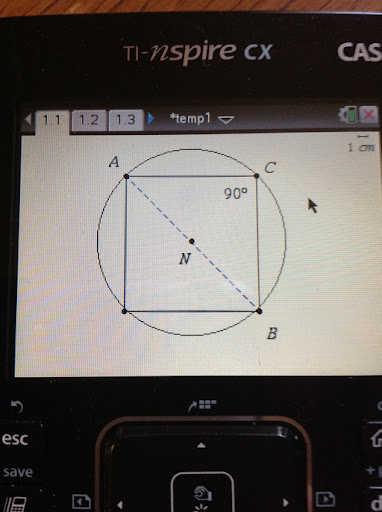Known: The radius, which is represented by line segment

AN
.
Goal: Find the dimension of one side of the square. Let the measure of the line segment AC be represent the measure of the side.

Let:
AB

represent the line segment which length is the diameter of the circle.

By the Pythagorean Theorem:

AC^2 + CB^2 = AB^2

Since AC = CB and AB = 2 AN,

2 AC^2 = (2 AN)^2
AC^2 = 2 AN^2
AC = AN √2

Take the positive root, as the negative root has negligible meaning.

The measure of the square's side is the radius times √2. (s = r √2)

Largest Circle in a Square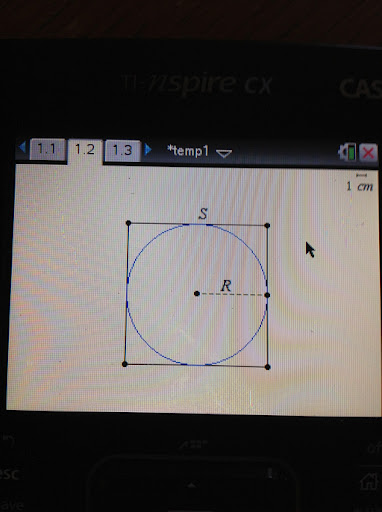Known: Radius of the circle, R.
Goal: Find the length of a side of the square, S.

Observe that the largest circle will fit when it's center is in the square's center. As the picture above indicates, some of the edge of the circle touches the square.

Therefore, S = 2 R

Largest Triangle in a Square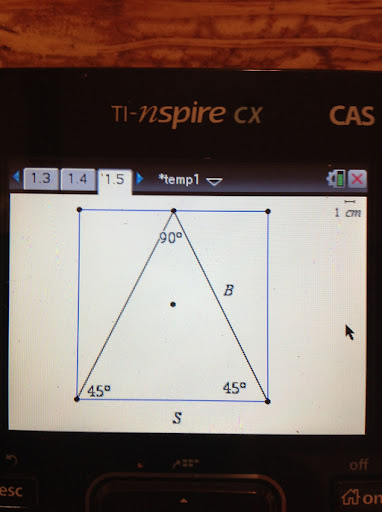Known: A square with each side measuring S.
Goal: The dimensions of the largest triangle.

If we want the largest triangle to fit in the square, it would make sense to make one of the sides to be of length S. It would also make sense to have the vertex of the triangle's two other sides at the other side of the square. This can be accomplished by a 45°-45°-90° triangle, not an equilateral triangle. (See the diagram above)

To solve for B, use trigonometry.

Observe that sin 45° = cos 45° = √2 / 2

Then:

√2 / 2 = B / S
B = (S √2) / 2

So our triangle would have the dimensions S × (S √2) / 2 × (S √2) / 2

Largest Square in an Equilateral Triangle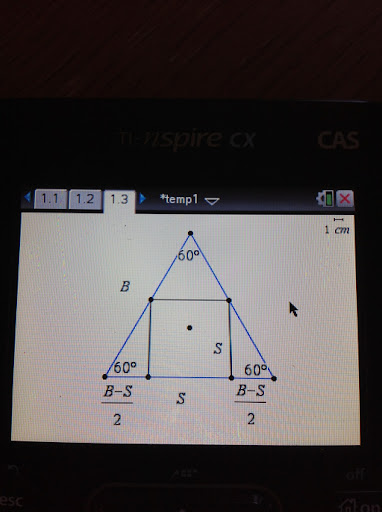Known: An equilateral triangle with sides of length B.
Goal: The length of the inscribed square, S.

Observe, by inspection, that the inscribed square is the largest when one of it's sides is touching the triangle's base. This allows for the largest S possible.

The measurement of the base is (B - S)/2 + S + (B - S)/2 = B.

We can find S by working with one of the two "mini right triangles" formed at the bottom of the triangle. A diagram of one of the "mini right triangles" is shown below: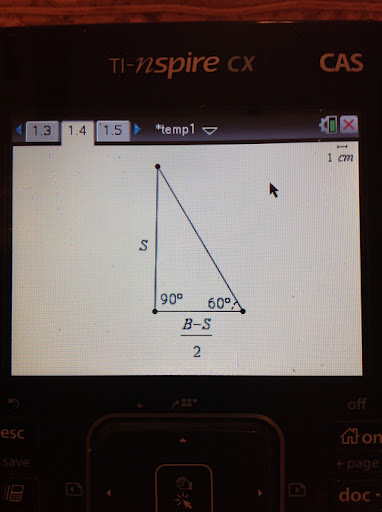Using trigonometry:

tan 60° = S / ((B - S) / 2)
√3 = (2S) / (B - S)
B √3 - S √3 = 2S

S = (B √3) / (2 + √3)

which is the desired result.

Largest Circle in an Equilateral TriangleI admit I have some difficulty with this one. In order for the circle to fit, the following have to be true:

1. The area of the triangle must be greater than the area of the circle.
2. The length of the triangle's side must be greater than twice the circle's radius.
3. The height of the triangle must be greater than twice the circle's radius.

Even obeying these rules did not guarantee an answer.

For me this is an open question. This may be one where numerical methods must be used. Any ideas, as always, are welcome.

Until then,

Eddie

This blog is property of Edward Shore. © 2012

## Monday, September 3, 2012

### Labor Day: Reference Angle, Construction Calculators, Why a Negative Times a Negative is Positive

Reference Angle

Each angle can be represented by a reference angle. The reference angle is determined by finding the smallest angle between the terminal ("ending side") side of the angle and the x-axis.

The reference angle is always positive and has the range 0° ≤ θ ≤ 90°.

Examples:
The reference angle of 135° is 45°.
The reference angle of 220° is 30°.
The reference angle of 329° is 31°.

This page by www.analyzemath.com gives examples and a method of finding the reference angle.

Math warehouse also gives a good explanation of reference angle.

Another way to get a reference angle is to use a scientific calculator. For most, if not all scientific calculators and math programs, the arcsin function (usually labeled sin^-1) gives the angle between -90° and 90°. On a scientific calculator, we can use the following keystroke sequence:

Angle [SIN] [SIN^-1] [ABS]

Or the command abs(asin(sin(x)). A graph of the reference angle is shown below. The angle in the graph is measure of radians.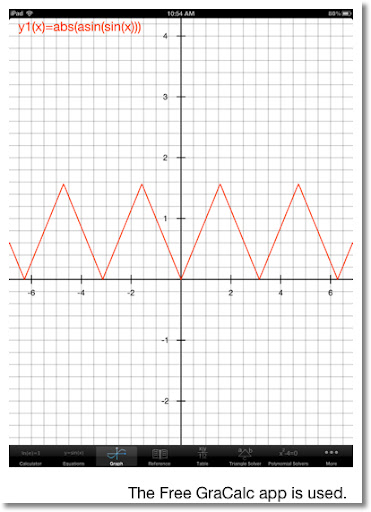Construction Calculators

I usually accompany my dad to trips to Home Depot and Lowes whenever he gets ideas for home improvement. The latest one was to build a fence. My dad and I often talk about construction and home improvement plans.

Calculated Industries is a company that makes specialized calculators that cover construction, real estate, hot rods, electronics, and even cooking and quilting. While I dare say the math can be done with a scientific and finance calculator, these calculators are good because (1) the keys are specialized and (2) they focus on a specialized area.

I managed to buy all three of calculators the stores have, which two of them are pictured below. The Materials Estimator is just what it says, it's main focus is estimate the materials needed, such as of tiles needed, gallons of paint, or my favorite, the fence materials. The Construction Master 5 focuses on roofs, stairs, and circular structures. My favorite feature of the construction calculators is the ability to calculate dimensional math in yards, feet, and inches with fractions (precision can be set to 1/64).

Whether a home improvement project is actually going to happen I am not sure (cost is a factor), but at the least these calculators are nice additions to my collection.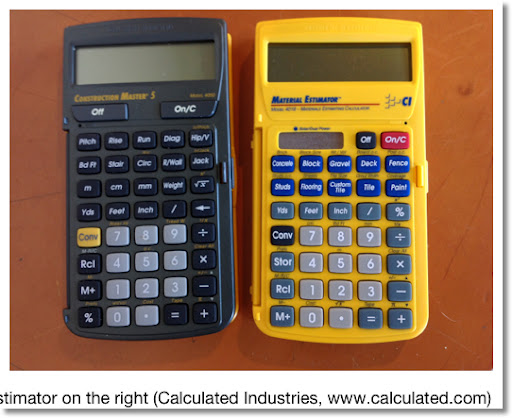Why a Negative Times a Negative is a Positive

We all heard the rule "A negative number times a negative number is a positive number". This line reminds me of the 1988 movie "Stand and Deliver". And yes, I had to look up the title because I remember the wrong name, but I remember Edward James Olmos as a teacher getting his classroom to chant the rule.

Confession: I am not a movie person, at all This may be strange considering I was born in Los Angeles blocks away from Hollywood. Sitting a dark room for two or more hours looking at a screen just doesn't do anything for me.

Anyway, I feel like for students to understand and fully grasp this concept, a nice short, concise explanation is needed.

Some resources tend to use an example such as:

-4 × -5
= -1 × 4 × -1 × 5
Using the Commutative Property for multiplication:
= -1 × -1 × 4 × 5
= -1 × -1 × 20
= 20.

Yet I feel this explanation is incomplete. There is no explanation of why -1 × -1 = 1.

I think a better way to show that a negative times a negative is positive is to consider the equation:

S = A × B + -A × B + -A × -B

Where S is the sum of the right hand expression, and A and B are positive numbers. (A > 0, B > 0).

We can find S in two ways. First, factor out a B in the first and second term of the right hand side:

S = A × B + -A × B + -A × -B
= (A + -A) × B + -A × -B
= 0 + -A × -B
= -A × -B

A second way is to factor -A on the second and third term on the right hand side:

S = A × B + -A × B + -A × -B
= A × B + -A × (B + -B)
= A × B + 0
= A × B

Note the following:
A > 0 and B > 0.
-A < 0 and -B < 0.
And A × B > 0, and S > 0.

Also, note S = A × B = -A × -B, and since S > 0, this implies -A × -B > 0.

There are many explanations that can be found on a web search either on a search engine or on YouTube. This one is my favorite because it is the most logical and it introduces logic and mathematical thinking to students.

That concludes this entry for today. Have a great Labor Day and I will talk to you soon!

Eddie

This blog is property of Edward Shore. © 2012

### HP 15C: Error Function and Lower Tail Normal Cumulative Function

HP 15C:   Error Function and Lower Tail Normal Cumulative Function Formulas Used  Error Function  erf(x) = 2 ÷ √π * ∫( e^(-t^2) dt, t = 0 t...